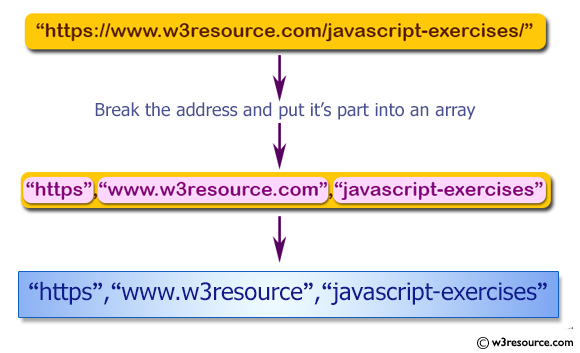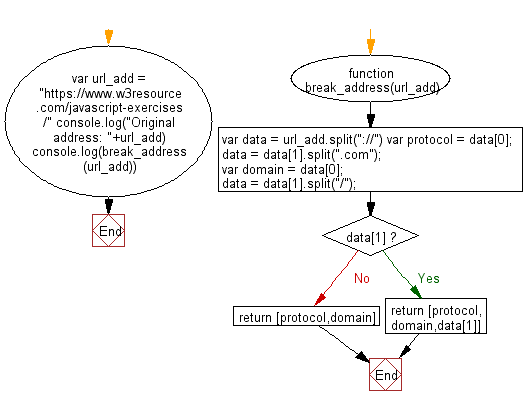# JavaScript: Break an address of an url and put it's part into an array

## JavaScript Basic: Exercise-144 with Solution

Write a JavaScript program to break an address of an url and put it's part into an array.

Note: url structure : ://.org[/] and there may be no part in the address.

Pictorial Presentation:Sample Solution:

HTML Code:

``````<!DOCTYPE html>
<html>
<meta charset="utf-8">
<meta name="viewport" content="width=device-width">
<title>Break an address of an url and put it's part into an array.</title>
<body>

</body>
</html>
```
```

JavaScript Code:

``````function break_address(url_add) {
var protocol = data;
data = data.split(".com");
var domain = data;
data = data.split("/");

if(data){
return [protocol,domain,data]
}

return [protocol,domain]
}

``````

Sample Output:

```Original address: https://www.w3resource.com/javascript-exercises/
["https","www.w3resource","javascript-exercises"]
```

Flowchart:ES6 Version:

``````function break_address(url_add) {
const protocol = data;
data = data.split(".com");
const domain = data;
data = data.split("/");

if(data){
return [protocol,domain,data]
}

return [protocol,domain]
}

``````

Live Demo:

See the Pen javascript-basic-exercise-144 by w3resource (@w3resource) on CodePen.

Improve this sample solution and post your code through Disqus

What is the difficulty level of this exercise?

Test your Programming skills with w3resource's quiz.

﻿

## JavaScript: Tips of the Day

Returns the sum of the powers of all the numbers from start to end (both inclusive)

Example:

```const sumPower = (end, power = 2, start = 1) =>
Array(end + 1 - start)
.fill(0)
.map((x, i) => (i + start) ** power)
.reduce((a, b) => a + b, 0);
console.log(sumPower(10)); // 385
console.log(sumPower(10, 3)); // 3025
console.log(sumPower(10, 3, 5)); // 2925
```

Output:

```385
3025
2925
```